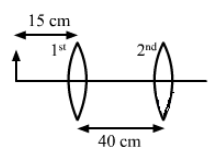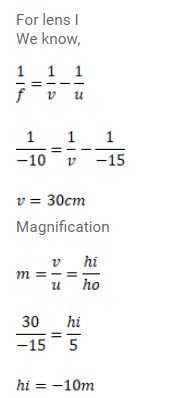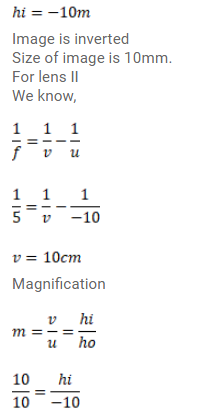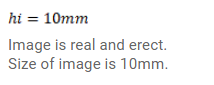# A 5 mm high pin is placed at a distance of 15cmQuestion:

A $5 \mathrm{~mm}$ high pin is placed at a distance of $15 \mathrm{~cm}$ from a convex lens focal length $10 \mathrm{~cm}$. A second lens of focal length $5 \mathrm{~cm}$ is placed $40 \mathrm{~cm}$ from the first lens and $55 \mathrm{~cm}$ from the pin. Find

(a) the position of the final image,

(b) its nature and

(c) its size.

Solution: